#Function Repository Resource:

# NInverseFourierSinTransform

Find a numerical approximation for an inverse Fourier sine transform

Contributed by: Wolfram Research
 ResourceFunction["NInverseFourierSinTransform"][expr,ω,t] gives a numerical approximation to the inverse Fourier sine transform of expr evaluated at the numerical value t, where expr is a function of ω.

## Details and Options

The numerical approximation to the inverse Fourier sine transform of expr is by default defined to be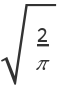NIntegrate[expr [ωt],{ω,0,}].
Different choices for the definition of the inverse Fourier sine transform can be specified using the option FourierParameters.
With the setting FourierParameters{a,b}, the inverse Fourier sine transform computed by ResourceFunction["NInverseFourierSinTransform"] is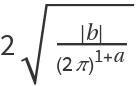NIntegrate[expr [bωt],{ω,0,}].
The parameter b in the setting FourierParameters{a,b} must be numeric.
In addition to the option FourierParameters, ResourceFunction["NInverseFourierSinTransform"] can also accept the options available to NIntegrate. These options are passed directly to NIntegrate.

## Examples

### Basic Examples

Numerical approximation for an inverse Fourier sine transform:

 In:=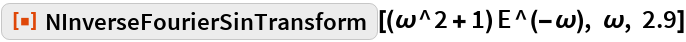Out=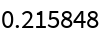Compare with the answer from symbolic evaluation:

 In:=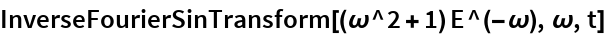Out=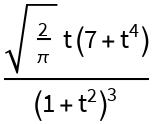In:=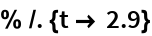Out=## Requirements

Wolfram Language 11.3 (March 2018) or above